2019-09-16 20:05:16 weixin_44225182 阅读数 2549
• ###### 机器学习算法实战——神秘奥妙的支持向量机

支持向量机算法是机器学习的重要算法，如今已应用在图像处理、 语音识别和自然语言处理等方面。本课程详细讲解支持向量机的原理、相关概念、 推导过程和代码实战。包括：Logistic函数、最大化间隔、凸二次优化、核函数、 数据中的噪声点处理等知识。最后用了手写字分类实例，详细讲解了相关的代码实战。

82 人正在学习 去看看 穆辉宇

椒盐噪声也称为脉冲噪声，是图像中经常见到的一种噪声，它是一种随机出现的白点或者黑点，可能是亮的区域有黑色像素或是在暗的区域有白色像素（或是两者皆有）。盐和胡椒噪声的成因可能是影像讯号受到突如其来的强烈干扰而产生、类比数位转换器或位元传输错误等。例如失效的感应器导致像素值为最小值，饱和的感应器导致像素值为最大值。

``````t=imread('a1.jpg');
subplot(1,2,1),imshow(t),title('原图');
t1=imnoise(t,'salt & pepper',0.1);
subplot(1,2,2),imshow(t1),title('加入噪声密度：0.1的椒盐噪声');
t2=imnoise(t,'salt & pepper',0.2);
figure,subplot(1,2,1),imshow(t2),title('加入噪声密度：0.2的椒盐噪声');
t3=imnoise(t,'salt & pepper',0.3);
subplot(1,2,2),imshow(t3),title('加入噪声密度：0.3的椒盐噪声');
``````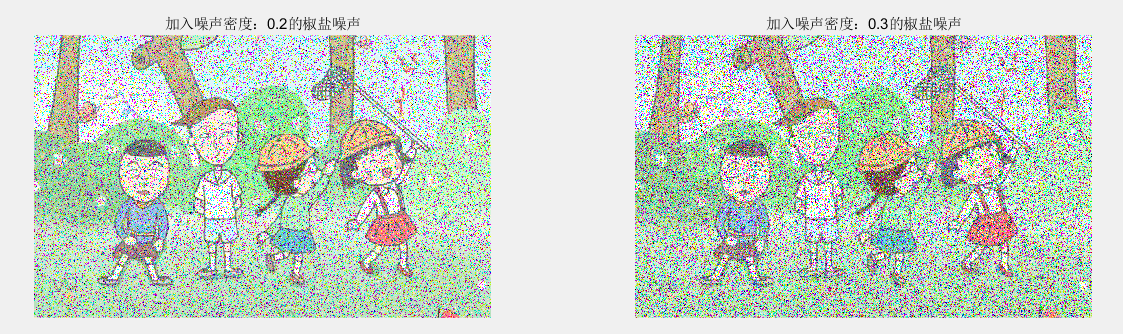• 噪声类型是 ‘salt & pepper’ 一定注意空格的位置 这里比较严格
• 当噪声类型是’salt & pepper’的时候，第三个参数的意思是噪声密度，比如0.1，那么总像素个数的10%为黑白点，当然是黑点还是白点都是随机的。

``````image=imread('a1.jpg');
[width,height,z]=size(image);

result2=image;
subplot(1,2,1)
imshow(image);
title('原图');

%k1、k2作为判断临界点
k1=0.2;
k2=0.2;
%rand(m,n)是随机生成m行n列的矩阵，每个矩阵元素都在0-1之间
%这里k都是0.2，所以小于k的元素在矩阵中为1，反之为0
a1=rand(width,height)<k1;
a2=rand(width,height)<k2;
%合成彩色图像
t1=result2(:,:,1);
t2=result2(:,:,2);
t3=result2(:,:,3);
%分成黑点 白点 随机
t1(a1&a2)=0;
t2(a1&a2)=0;
t3(a1&a2)=0;
t1(a1& ~a2)=255;
t2(a1& ~a2)=255;
t3(a1& ~a2)=255;
result2(:,:,1)=t1;
result2(:,:,2)=t2;
result2(:,:,3)=t3;
subplot(1,2,2)
imshow(result2);
title('加椒盐噪声后');
``````1.c=rand（10,10）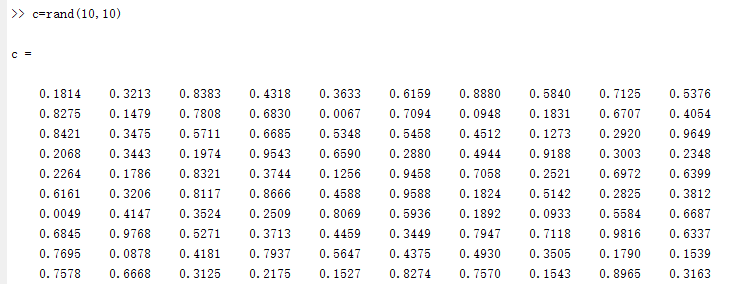2.a=c<0.2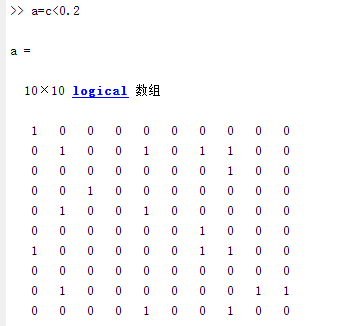3.b=rand（10,10）<0.2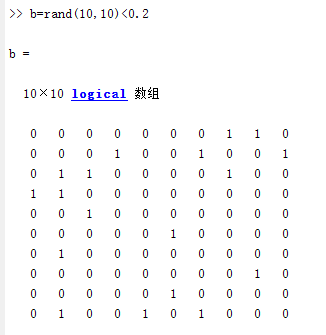4.在分别a&b a&~b
a&b：a和b队员元素都为1的时候，则结果为1，反正为0. 显示白点或者黑点
a&~b:a为1 b为0时，结果为1 显示黑点或者白点

## 更多

2019-05-05 09:51:00 m0_37992521 阅读数 503
• ###### 机器学习算法实战——神秘奥妙的支持向量机

支持向量机算法是机器学习的重要算法，如今已应用在图像处理、 语音识别和自然语言处理等方面。本课程详细讲解支持向量机的原理、相关概念、 推导过程和代码实战。包括：Logistic函数、最大化间隔、凸二次优化、核函数、 数据中的噪声点处理等知识。最后用了手写字分类实例，详细讲解了相关的代码实战。

82 人正在学习 去看看 穆辉宇

## 椒盐噪声

1. 什么是椒盐噪声
椒盐噪声，就是椒噪声和盐噪声的混合噪声。其中，椒噪声的椒即是黑胡椒之意，在图像中表现为黑色点斑；而盐噪声则是取自食盐，在图像中表现为白色点状。一般两种噪声在图像中混合出现，表现为黑白混杂。
2. 如何添加椒盐噪声
因为椒噪声表现为黑色，属于低灰度图像；盐噪声表现为白色，属于高灰度图像。利用这种特性，可以在图像中随机选取像素点赋值为0或者255，通常为了便于人眼分辨，随机赋值为255.
3. 如何去除椒盐噪声
椒盐噪声的像素值为0或者255，用中值滤波的方法处理效果非常好。中值滤波是在对一个区域的像素值进行大小排序，取其中值代表这一区域的像素值。因此对于消弱高低频分量对图像的影响的效果非常明显。
opencv实现程序：https://blog.csdn.net/m0_37992521/article/details/89840367

2017-08-03 11:49:49 u010368556 阅读数 13409
• ###### 机器学习算法实战——神秘奥妙的支持向量机

支持向量机算法是机器学习的重要算法，如今已应用在图像处理、 语音识别和自然语言处理等方面。本课程详细讲解支持向量机的原理、相关概念、 推导过程和代码实战。包括：Logistic函数、最大化间隔、凸二次优化、核函数、 数据中的噪声点处理等知识。最后用了手写字分类实例，详细讲解了相关的代码实战。

82 人正在学习 去看看 穆辉宇

http://blog.csdn.net/qq_34784753/article/details/69379135

1.椒盐噪声

椒盐噪声也称为脉冲噪声，是图像中经常见到的一种噪声，它是一种随机出现的白点或者黑点，可能是亮的区域有黑色像素或是在暗的区域有白色像素（或是两者皆有）。盐和胡椒噪声的成因可能是影像讯号受到突如其来的强烈干扰而产生、类比数位转换器或位元传输错误等。例如失效的感应器导致像素值为最小值，饱和的感应器导致像素值为最大值。图像模拟添加椒盐噪声是通过随机获取像素点并设置为高亮度点和低灰度点来实现的

2.高斯噪声

高斯噪声是指高绿密度函数服从高斯分布的一类噪声。特别的，如果一个噪声，它的幅度分布服从高斯分布，而它的功率谱密度有事均匀分布的，则称这个噪声为高斯白噪声。高斯白噪声二阶矩不相关，一阶矩为常数，是指先后信号在时间上的相关性。高斯噪声包括热噪声和三里噪声。高斯噪声万有由它的事变平均值和两瞬时的协方差函数来确定，若噪声是平稳的，则平均值与时间无关，而协方差函数则变成仅和所考虑的两瞬时之差有关的相关函数，在意义上它等同于功率谱密度。高斯早生可以用大量独立的脉冲产生，从而在任何有限时间间隔内，这些脉冲中的每一个买充值与所有脉冲值得总和相比都可忽略不计。

根据Box-Muller变换原理，建设随机变量U1、U2来自独立的处于(0,1)之间的均匀分布，则经过下面两个式子产生的随机变量Z0，Z1服从标准高斯分布。```#include <cstdlib>
#include <iostream>
#include <opencv2\core\core.hpp>
#include <opencv2\highgui\highgui.hpp>
#include <opencv2\imgproc\imgproc.hpp>

using namespace cv;
using namespace std;

double generateGaussianNoise(double m, double sigma);
Mat addSaltNoise(const Mat srcImage, int n);

int main()
{
if (!srcImage.data)
{
cout << "读入图像有误！" << endl;
system("pause");
return -1;
}
imshow("原图像", srcImage);
imshow("添加椒盐噪声的图像", dstImage1);
imshow("添加高斯噪声的图像", dstImage2);
//存储图像
imwrite("salt_pepper_Image.jpg", dstImage1);
imwrite("GaussianNoise_Image.jpg", dstImage2);
waitKey();
return 0;
}

Mat addSaltNoise(const Mat srcImage, int n)
{
Mat dstImage = srcImage.clone();
for (int k = 0; k < n; k++)
{
//随机取值行列
int i = rand() % dstImage.rows;
int j = rand() % dstImage.cols;
//图像通道判定
if (dstImage.channels() == 1)
{
dstImage.at<uchar>(i, j) = 255;       //盐噪声
}
else
{
dstImage.at<Vec3b>(i, j) = 255;
dstImage.at<Vec3b>(i, j) = 255;
dstImage.at<Vec3b>(i, j) = 255;
}
}
for (int k = 0; k < n; k++)
{
//随机取值行列
int i = rand() % dstImage.rows;
int j = rand() % dstImage.cols;
//图像通道判定
if (dstImage.channels() == 1)
{
dstImage.at<uchar>(i, j) = 0;     //椒噪声
}
else
{
dstImage.at<Vec3b>(i, j) = 0;
dstImage.at<Vec3b>(i, j) = 0;
dstImage.at<Vec3b>(i, j) = 0;
}
}
return dstImage;
}
//生成高斯噪声
double generateGaussianNoise(double mu, double sigma)
{
//定义小值
const double epsilon = numeric_limits<double>::min();
static double z0, z1;
static bool flag = false;
flag = !flag;
//flag为假构造高斯随机变量X
if (!flag)
return z1 * sigma + mu;
double u1, u2;
//构造随机变量
do
{
u1 = rand() * (1.0 / RAND_MAX);
u2 = rand() * (1.0 / RAND_MAX);
} while (u1 <= epsilon);
//flag为真构造高斯随机变量
z0 = sqrt(-2.0*log(u1))*cos(2 * CV_PI*u2);
z1 = sqrt(-2.0*log(u1))*sin(2 * CV_PI*u2);
return z0*sigma + mu;
}

//为图像添加高斯噪声
{
Mat dstImage = srcImag.clone();
int channels = dstImage.channels();
int rowsNumber = dstImage.rows;
int colsNumber = dstImage.cols*channels;
//判断图像的连续性
if (dstImage.isContinuous())
{
colsNumber *= rowsNumber;
rowsNumber = 1;
}
for (int i = 0; i < rowsNumber; i++)
{
for (int j = 0; j < colsNumber; j++)
{
//添加高斯噪声
int val = dstImage.ptr<uchar>(i)[j] +
generateGaussianNoise(0, 2.235) * 32;
if (val < 0)
val = 0;
if (val>255)
val = 255;
dstImage.ptr<uchar>(i)[j] = (uchar)val;
}
}
return dstImage;
}```

2019-01-30 01:37:12 qq_42505705 阅读数 643
• ###### 机器学习算法实战——神秘奥妙的支持向量机

支持向量机算法是机器学习的重要算法，如今已应用在图像处理、 语音识别和自然语言处理等方面。本课程详细讲解支持向量机的原理、相关概念、 推导过程和代码实战。包括：Logistic函数、最大化间隔、凸二次优化、核函数、 数据中的噪声点处理等知识。最后用了手写字分类实例，详细讲解了相关的代码实战。

82 人正在学习 去看看 穆辉宇原图　　　　　　　　　　　　　　　　　　加椒盐噪声后的图像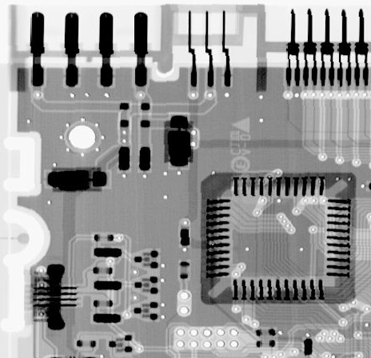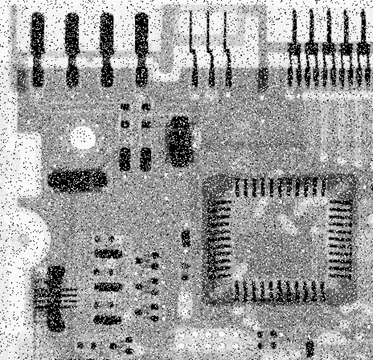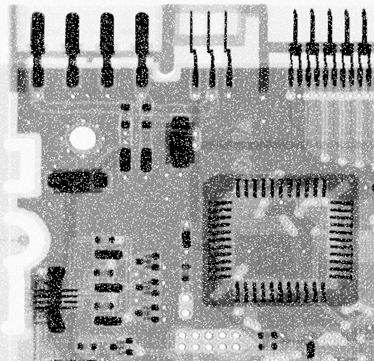``````import numpy as np
import cv2

#定义添加椒盐噪声的函数
def SaltAndPepperNoise(img,percetage):
SP_NoiseImg=img
SP_NoiseNum=int(percetage*img.shape*img.shape)
for i in range(SP_NoiseNum):
randX=np.random.random_integers(0,img.shape-1)
randY=np.random.random_integers(0,img.shape-1)
if np.random.random_integers(0,1)==0:
SP_NoiseImg[randX,randY]=0   #0为胡椒噪声
else:
SP_NoiseImg[randX,randY]=255    #1为盐粒噪声
return SP_NoiseImg

#定义添加高斯噪声的函数
G_Noiseimg = image
G_NoiseNum=int(percetage*image.shape*image.shape)
for i in range(G_NoiseNum):
temp_x = np.random.randint(0,G_Noiseimg.shape)
temp_y = np.random.randint(0,G_Noiseimg.shape)
G_Noiseimg[temp_x][temp_y] = 255
return G_Noiseimg

def main():
cv2.namedWindow("Original image")
cv2.imshow("Original image", img)
cv2.imshow("grayimage", grayImg)

gauss_noiseImage = addGaussianNoise(grayImg, 0.1)  # 添加10%的高斯噪声
cv2.imwrite(r"C:\Users\xxx\Desktop\GaussianNoise Image1.jpg", gauss_noiseImage)

SaltAndPepper_noiseImage = SaltAndPepperNoise(grayImg, 0.1)  # 添加10%的椒盐噪声
cv2.imwrite(r"C:\Users\xxx\Desktop\SaltAndPepper_noiseImage1.jpg", gauss_noiseImage)

cv2.waitKey(0)
cv2.destroyAllWindows()

main()

``````

``````mbImg = cv2.medianBlur(noise_im,3)
``````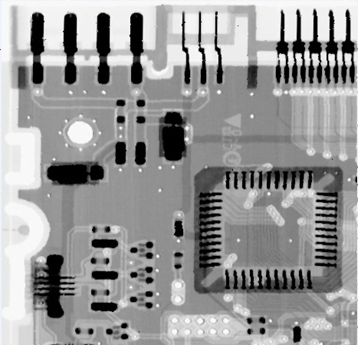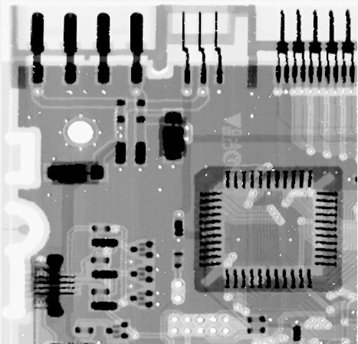``````meanImg = cv2.blur(noise_im,(3,3))
``````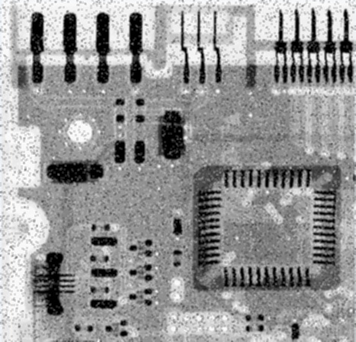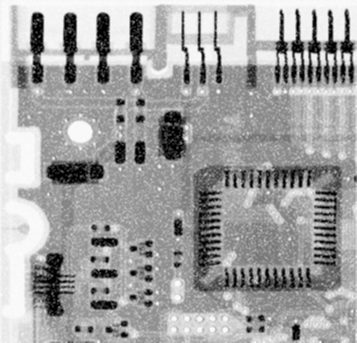``````Img_GaussianBlur=cv2.GaussianBlur(noise_im,(3,3),0)
``````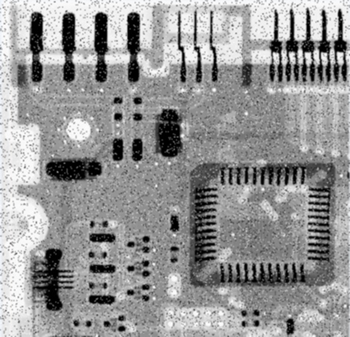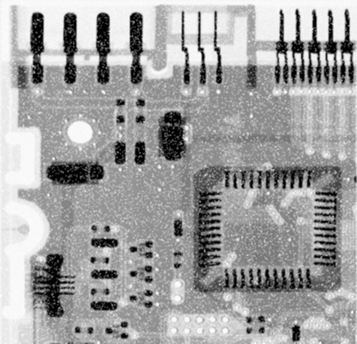``````img_bilateralFilter=cv2.bilateralFilter(noise_im,40,75,75)
``````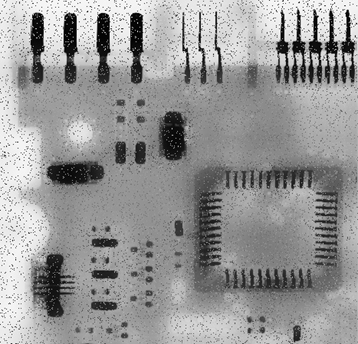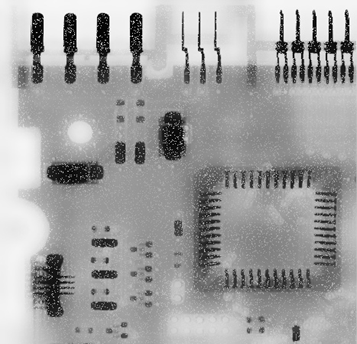2013-11-30 14:50:00 u012999424 阅读数 4442
• ###### 机器学习算法实战——神秘奥妙的支持向量机

支持向量机算法是机器学习的重要算法，如今已应用在图像处理、 语音识别和自然语言处理等方面。本课程详细讲解支持向量机的原理、相关概念、 推导过程和代码实战。包括：Logistic函数、最大化间隔、凸二次优化、核函数、 数据中的噪声点处理等知识。最后用了手写字分类实例，详细讲解了相关的代码实战。

82 人正在学习 去看看 穆辉宇

图像噪声是影响人们接受图像信息的因素，常见的噪声有高斯噪声和椒盐噪声。因为最近课程要求，做一个图像恢复的Project，所以掌握了给图像添加噪声以及去除噪声的方法。

高斯噪声是大量具有正太分布的随机变量性质的值加到原图像造成的，要给图像添加高斯噪声，其实问题就是怎么产生正太分布随机变量。首先用Randdom对象的NextDouble产生两个0-1之间的随机变量r1，r2，计算

`           double result = Math.Sqrt((-2) * Math.Log(r2)) * Math.Sin(2 * Math.PI * r1);`

得到的result就是具有均值0，方差1的正太分布随机变量。这是box-muller方法，算法推导很复杂，但实现却很方便。因为对图像添加高斯噪声的时候，对于每一个像素都需要产生r1，r2以便得到噪声，这就需要快速大量地产生随机变量，一开始我发现产生的随机变量总是连续相同，也就是说在很短的时间内产生的随机数是相同的，因为毕竟C#Random产生的是伪随机数，是通过一定的算法算出来的，而且有依据“种子”来计算，默认情况下是依据电脑此时时间来计算，但是当快速大量此类的随机数时，会出现连续产生相同随机数的情况，因为电脑时间不是一个很好的“种子”。所以我的程序里用了这样的办法：

```        static int GetRandomSeed( ) //产生随机种子
{
byte[] bytes = new byte;
System.Security.Cryptography.RNGCryptoServiceProvider rng = new System.Security.Cryptography.RNGCryptoServiceProvider( );
rng.GetBytes( bytes );
return BitConverter.ToInt32( bytes , 0 );
}

public double GaussNiose1()//用box muller的方法产生均值为0，方差为1的正太分布随机数
{
// Random ro = new Random(10);
// long tick = DateTime.Now.Ticks;
Random ran = new Random(GetRandomSeed());
// Random rand = new Random();
double r1 = ran.NextDouble();
double r2 = ran.NextDouble();
double result = Math.Sqrt((-2) * Math.Log(r2)) * Math.Sin(2 * Math.PI * r1);
return result;//返回随机数
}```

这样问题就解决了，可以短时间快速产生随机数。

添加椒盐噪声的方法如下：

``` private void AddSalt(object sender, EventArgs e)
{
if (textBox12.Text != "" && textBox13.Text != "")
{
// Bitmap pic = (Bitmap)Bitmap.FromFile(filename, false);
Bitmap pic = new Bitmap(pictureBox2.Image, WI, HE);
double Pa = Convert.ToDouble(textBox12.Text);//接受输入的Pa
double Pb = Convert.ToDouble(textBox13.Text);//接受输入的Pb
double P = Pb / (1 - Pa);//程序要,为了得到一个概率Pb事件
int width = pic.Width;
int height = pic.Height;
Random rand = new Random();
for (int i = 0; i < height; i++)
{
for (int j = 0; j < width; j++)
{
int gray;
int noise = 1;
double probility = rand.NextDouble();
if (probility < Pa)
{
noise = 255;//有Pa概率 噪声设为最大值
}
else
{
double temp = rand.NextDouble();
if (temp < P)//有1 - Pa的几率到达这里，再乘以 P ，刚好等于Pb
noise = 0;
}
if (noise != 1)
{
gray = noise;
}
else gray = pic.GetPixel(j, i).R;
Color color = Color.FromArgb(gray, gray, gray);
pic.SetPixel(j, i, color);
}
}
Form2 f2 = new Form2();
f2.change_size(pic);
f2.Setname("图像添加椒盐噪声之后的图像，需要还原的话请先保存然后再打开^_^");
f2.Show();
}
else
{
MessageBox.Show("请先输入Pa和Pb^_^");
}
}```

对于每一个像素，取出周围九个像素，存到filter，然后对这个数组进行排序，最后取到中间值作为目标值。下面程序是在取值的时候就同时进行排序，采用插入排序法。

```   private void MedianFilter(object sender, EventArgs e)
{
Bitmap pic = new Bitmap(pictureBox2.Image, WI, HE);
int width = pic.Width;
int height = pic.Height;
int[,] resultPic = new int[height, width];
int index;
int[] filter = new int;
for (int i = 0; i < height; i++)
{
for (int j = 0; j < width; j++)
{
index = 0;
int nowGray;
for (int ii = -1; ii < 2; ii++)
{
for (int jj = -1; jj < 2; jj++)
{
if (j + jj >= 0 && j + jj < width && i + ii >= 0 && i + ii < height)
{
nowGray = pic.GetPixel(j + jj, i + ii).R;
}
else { nowGray = 0; }
if (index == 0) { filter[index] = nowGray; index++; }
else
{
if (nowGray >= filter[index-1])
{
filter[index++] = nowGray;
}
else
{
int current = index-1;
while (current > 0 && filter[current] > nowGray)
{
filter[current + 1] = filter[current];
current--;
}
filter[current+1] = nowGray;
index++;
}

}

}
}
resultPic[i,j] = filter;
// int temp = filter;
//  Color color = Color.FromArgb(temp, temp, temp);
// pic.SetPixel(j, i, color);
}
}
for (int i = 0; i < height; i++)
{
for (int j = 0; j < width; j++)
{
int temp = resultPic[i,j];
Color color = Color.FromArgb(temp, temp, temp);
pic.SetPixel(j, i, color);
}
}
Form2 f2 = new Form2();
f2.change_size(pic);
f2.Setname("中值滤波之后的图像");
f2.Show();
}```